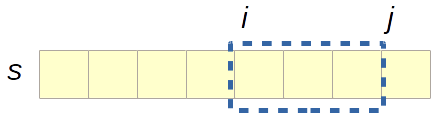# Programming-Idioms# Idiom #38 Extract a substring

Find substring t consisting in characters i (included) to j (excluded) of string s.
Character indices start at 0 unless specified otherwise.
Make sure that multibyte characters are properly handled.``my \$chunk = substr("now is the time", \$i, \$j);``
``T : String := S (I .. J - 1);``
``````#include <stdlib.h>
#include <string.h>``````
``````char *t=malloc((j-i+1)*sizeof(char));
strncpy(t,s+i,j-i);``````
``(def t (subs s i j))``
``#include <string>``
``auto t = s.substr(i, j-i);``
``var t = s.Substring(i, j - i);``
``auto t = s[i .. j];``
``var t = s.substring(i, j);``
``t = String.slice(s, i..j-1)``
``T = string:sub_string(I, J-1).``
``````character(len=:), allocatable :: t

t = s(i:j-1)
``````
``t := string([]rune(s)[i:j])``
``def t = s[i..<j]``
``````t = drop i (take j s)
``````
``let t = s.slice(i, j);``
``let t = s.substring(i, j);``
``````String t = s.substring(i,j);
``````
``val t = s.substring(i, j)``
``````(setf u (subseq s i j))
``````
``@import Foundation;``
``t=[s substringWithRange:NSMakeRange(i,j-i)]``
``\$t = mb_substr(\$s, \$i, \$j-\$i, 'UTF-8');``
``uses Sysutils;``
``t := s.Substring(i,j-i);``
``t := copy(s,i+1,j-i);``
``t = s[i:j]``
``t = s[i..j-1] ``
``````extern crate unicode_segmentation;
use unicode_segmentation::UnicodeSegmentation;``````
``let t = s.graphemes(true).skip(i).take(j - i).collect::<String>();``
``use substring::Substring;``
``let t = s.substring(i, j);``
``(define t (substring s i j))``
``t := s copyFrom: i to: j - 1.``
``Dim t As String = s.Substring(i,j)``

programming-idioms.org